## Overview

Arm Neon technology is a 64-bit or 128-bit hybrid Single Instruction Multiple Data (SIMD) architecture that is designed to accelerate the performance of multimedia and signal processing applications. These applications include the following:

• Video encoding and decoding
• Audio encoding and decoding
• 3D graphics processing
• Speech processing
• Image processing

This guide provides information about how to write SIMD code for Neon using assembly language. This guide is written for anyone wanting to learn more about the Armv8-A instruction set architecture. The following readers should find the information particularly useful:

• Tools developers
• Low-level SoC programmers, such as firmware, device driver, or android kernel developers
• Programmers who want to optimize libraries or applications for an Arm-based target device
• Very keen Raspberry Pi enthusiasts

This guide covers getting started with Neon, using it efficiently, and hints and tips for more experienced coders. Specifically, this guide deals with the following subject areas:

• Memory operations, and how to use the flexible load and store instructions.
• Using the permutation instructions to deal with load and store leftovers.
• Using Neon to perform an example data processing task, matrix multiplication.
• Shifting operations, using the example of converting image data formats.

## Load and store - example RGB conversion

This section considers an example task of converting RGB data to BGR color data.

In a 24-bit RGB image, the pixels are arranged in memory as R, G, B, R, G, B, and so on. You want to perform a simple image-processing operation, like switching the red and blue channels. How can you do this efficiently using Neon?

Using a load that pulls RGB data items sequentially from memory into registers makes swapping the red and blue channels awkward.

Consider the following instruction, which loads RGB data one byte at a time from memory into consecutive lanes of three Neon registers:

`LD1 { V0.16B, V1.16B, V2.16B }, [x0]`

The following diagram shows the operation of this instruction: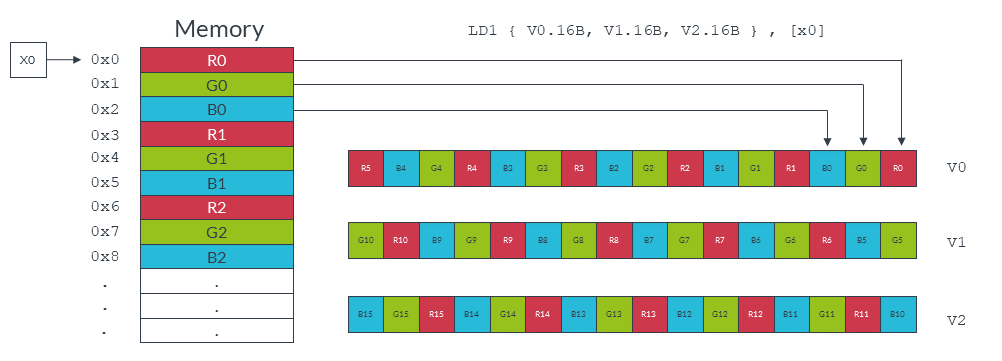Code to swap channels based on this input would be complicated. We would need to mask different lanes to obtain the different color components, then shift those components and recombine. The resulting code is unlikely to be efficient.

Neon provides structure load and store instructions to help in these situations. These instructions pull in data from memory and simultaneously separate the loaded values into different registers. For this example, you can use the `LD3` instruction to separate the red, green, and blue data values into different Neon registers as they are loaded:

`LD3 { V0.16B, V1.16B, V2.16B }, [x0]`

The following diagram shows how the above instruction separates the different data channels: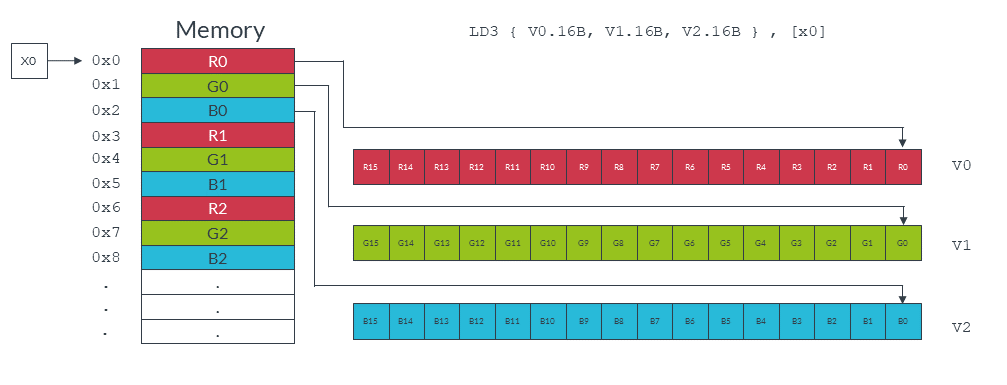The red and blue values can now be switched easily using the MOV instruction to copy the entire vector. Finally, we write the data back to memory, with reinterleaving, using the ST3 store instruction.

A single iteration of this RGB to BGR switch can be coded as follows:

```LD3  { V0.16B, V1.16B, V2.16B }, [x0], #48  // 3-way interleaved load from
// by 48
MOV    V3.16B, V0.16B                       // Swap V0 -> V3
MOV    V0.16B, V2.16B                       // Swap V2 -> V0
MOV    V2.16B, V3.16B                       // Swap V3 -> V2
// (net effect is to swap V0 and V2)
ST3  { V0.16B, V1.16B, V2.16B }, [x1], #48  // 3-way interleaved store to address
// in X1, post-incremented by 48```

Each iteration of this code does the following:

• Loads from memory 16 red bytes into `V0`, 16 green bytes into `V1`, and 16 blue bytes into `V2`.
• Increments the source pointer in `X0` by 48 bytes ready for the next iteration. The increment of 48 bytes is the total number of bytes that we read into all three registers, so 3 x 16 bytes in total.
• Swaps the vector of red values in `V0` with the vector of blue values in `V2`, using `V3` as an intermediary.
• Stores the data in `V0`, `V1`, and `V2` to memory, starting at the address that is specified by the destination pointer in `X1`, and increments the pointer.

## Load and store - data structures

Neon structure load instructions read data from memory into 64-bit Neon registers, with optional deinterleaving.

Structure store instructions work similarly, reinterleaving data from registers before writing it to memory, as shown in the following diagram: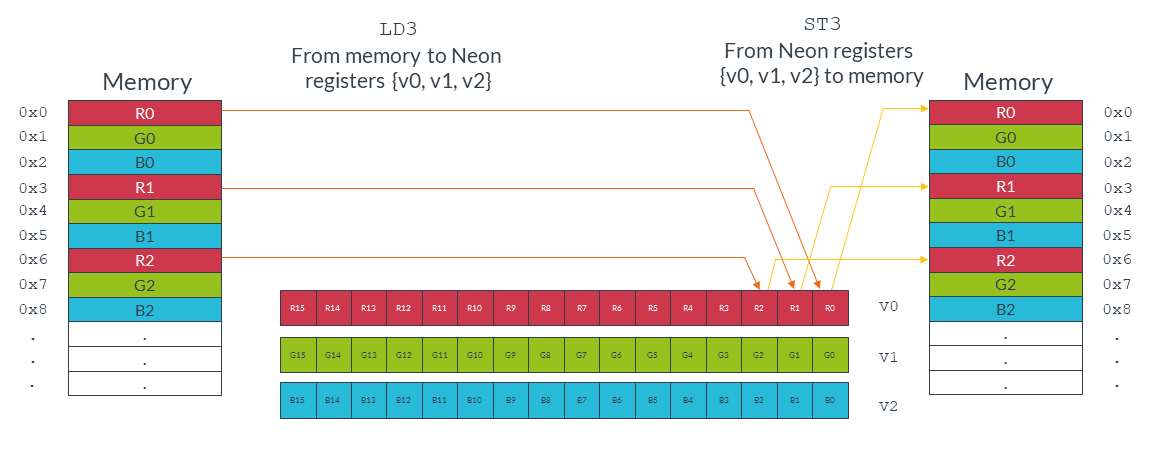## Syntax

The following diagram shows the general syntax of the structure load and store instructions: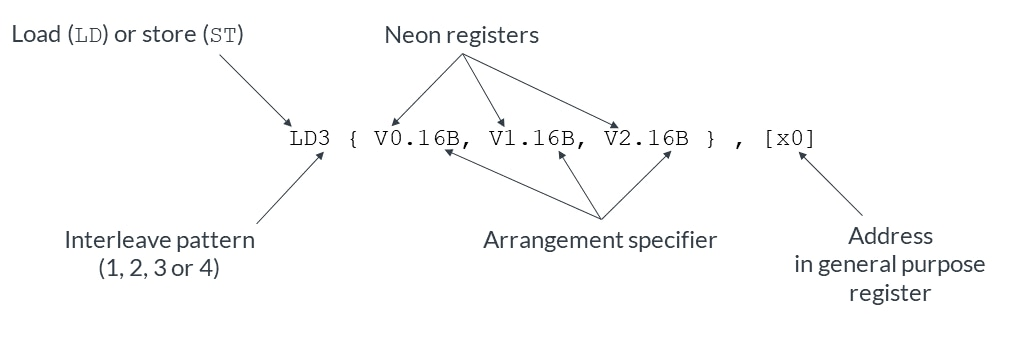This instruction syntax has the following format:

• An instruction mnemonic, with two parts:
• The operation, either `LD` for loads or `ST` for stores.
• A numeric interleave pattern specifying the number of elements in each structure.
• A set of 64-bit Neon registers to be read or written. A maximum of four registers can be listed, depending on the interleave pattern. Each entry in the set of Neon registers has two parts:
• The Neon register name, for example `V0`.
• An arrangement specifier. This indicates the number of bits in each element and the number of elements that can fit in the Neon vector register. For example, `16B` indicates that each element is one byte (`B`), and each vector is a 128-bit vector containing 16 elements.
• A general-purpose register containing the location to access in memory. The address can be updated after the access.

## Interleave pattern

Neon provides instructions to load and store interleaved structures containing from one to four equally sized elements. Elements are the standard Neon-supported widths of 8 (B), 16 (H), 32 (S), or 64 (D) bits.

• `LD1` is the simplest form. It loads one to four registers of data from memory, with no deinterleaving. You can use `LD1` to process an array of non-interleaved data.
• `LD2` loads two or four registers of data, deinterleaving even and odd elements into those registers. You can use `LD2` to separate stereo audio data into left and right channels.
• `LD3` loads three registers and deinterleaves. You can use `LD3` to split RGB pixel data into separate color channels.
• `LD4` loads four registers and deinterleaves. You can use `LD4` to process ARGB image data.

The store instructions `ST1`, `ST2`, `ST3`, and `ST4` support the same options, but interleave the data from registers before writing them to memory.

## Element types

Loads and stores interleave elements based on the size that is specified to the instruction.

For example, consider the following instruction:

`LD2 {V0.8H, V1.8H}, [X0]`

This instruction loads two Neon registers with deinterleaved data starting from the memory address in `X0`. The `8H` in the arrangement specifier indicates that each element is a 16-bit halfword (`H`), and each Neon register is loaded with eight elements. This instruction therefore results in eight 16-bit elements in the first register `V0`, and eight 16-bit elements in the second register `V1`. Adjacent pairs (even and odd) are separated to each register, as shown in the following diagram: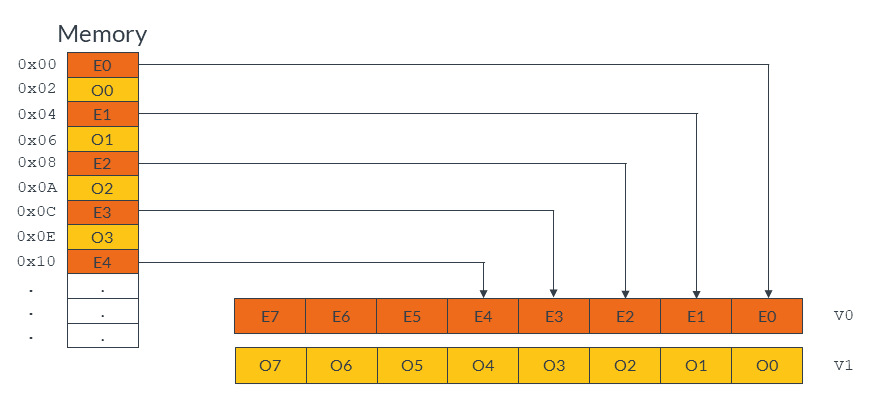The following instruction uses the arrangement specifier `4S`, changing the element size to 32-bits:

`LD2 {V0.4S, V1.4S}, [X0]`

Changing the element size to 32-bits loads the same amount of data, but now only four elements make up each vector, as shown in the following diagram: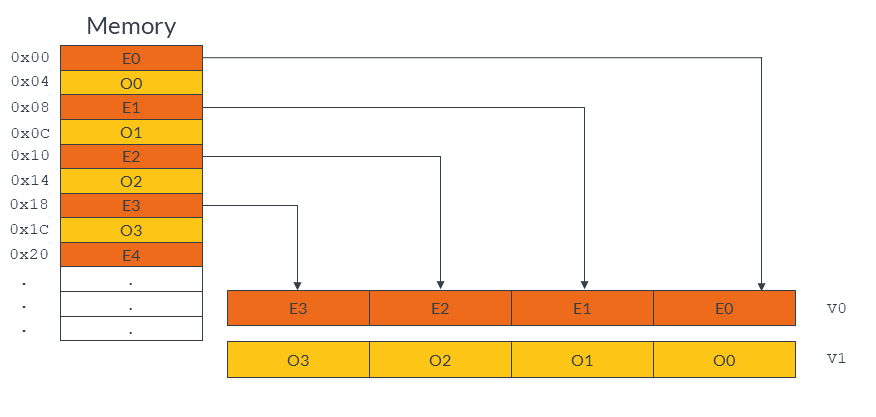Element size also affects endianness handling. In general, if you specify the correct element size to the load and store instructions, bytes are read from memory in the appropriate order. This means that the same code works on little-endian systems and big-endian systems.

Finally, element size has an impact on pointer alignment. Alignment to the element size generally gives better performance, and it might be a requirement of your target operating system. For example, when loading 32-bit elements, align the address of the first element to at least 32-bits.

## Single or multiple elements

In addition to loading multiple elements, structure loads can also read single elements from memory with deinterleaving. Data can either be replicated to all lanes of a Neon register, or inserted into a single lane, leaving the other lanes intact.

For example, the following instruction loads a single three-element data structure from the memory address pointed to by `X0`, then replicates that data into all lanes of three Neon registers:

`LD3R  { V0.16B, V1.16B, V2.16B } , [x0] `

The following diagram shows the operation of this instruction:By contrast, the following instruction loads a single three-element data structure into a single lane of three Neon registers, leaving the other lanes intact:

`LD3 { V0.B, V1.B, V2.B } , [x0]`

The following diagram shows the operation of this instruction. This form of the load instruction is useful when you need to construct a vector from data scattered in memory.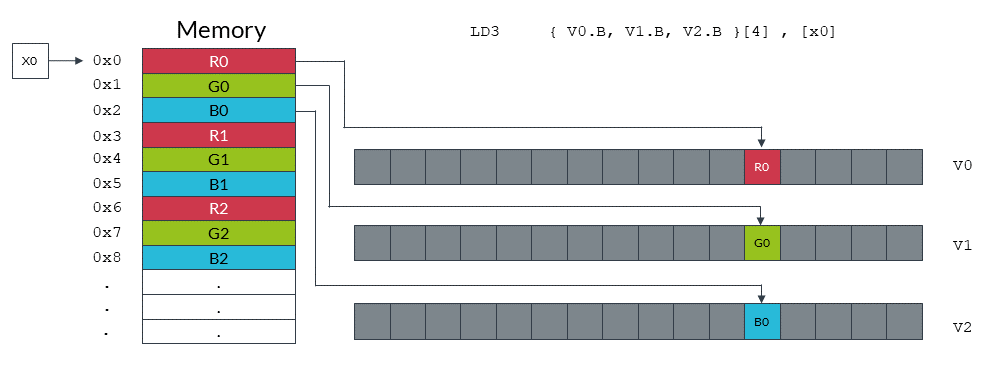Stores are similar, providing support for writing single or multiple elements with interleaving.

• Register (no offset): `[Xn]`

This is the simplest form. Data is loaded addition stored to the address that is specified by `Xn`.

• Register with post-index, immediate offset: `[Xn], #imm`

Use this form to update the pointer in `Xn` after loading or storing, ready to load or store the next elements.

The immediate increment value `#imm` must be equal to the number of bytes that is read or written by the instruction.

For example, the following instruction loads 48 bytes of data, using three registers, each containing 16 x 1 byte data elements. This means that the immediate increment is 48:

`LD3 { V0.16B, V1.16B, V2.16B }, [x0], #48`

However, the next example loads 32 bytes of data, using two registers, each containing 2 x  8 byte data elements. This means that the immediate increment is 32:

`LD2     { V0.2D, V1.2D}, [x0], #32`
• Register with post-index, register offset: `[Xn], Xm`

After the memory access, increment the pointer by the value in register `Xm`. This form is useful when reading or writing groups of elements that are separated by fixed widths, for example when reading a vertical line of data from an image.

## Other types of loads and stores

This guide only deals with structure loads and stores. However, Neon also provides other types of load and store instruction, including:

• `LDR` and `STR` to load and store single Neon registers.
• `LDP` and `STP` to load or store pairs of Neon registers.

For more details on supported load and store operations, see the Arm Architecture Reference Manual.

Detailed cycle timing information for the instructions can be found in the Technical Reference Manual for each core.

## Load and store - leftovers

A common situation when coding for Neon is dealing with input data that is not an exact multiple of the number of lanes in the vector register.

For example, consider an input array that contains 21 data elements, each of which is a 16-bit integer. You want to use Neon to process the data in this array. Neon registers are 128 bits wide, so can process eight lanes of 16-bit data at a time. In two iterations, your Neon code can process 16 (2 x 8) data elements. However, this leaves five leftover data elements to process in the final iteration. These five leftover data elements are not enough to completely fill a Neon register.

There are three approaches that you can take to handle these leftovers. Which method to choose depends on your requirements. The three approaches are as follows, with the fastest approach listed first:

If you can change the size of the arrays, you can increase the length of the array to the next multiple of the vector size using padding elements. This allows you to read and write beyond the end of your data without corrupting adjacent storage.

In our example with 21 data elements, increasing the array size to 24 elements allows the third iteration to complete without potential data corruption.

The following diagram shows how the three iterations load eight data elements into the Neon register. The final iteration loads the three padding elements along with the final five array values: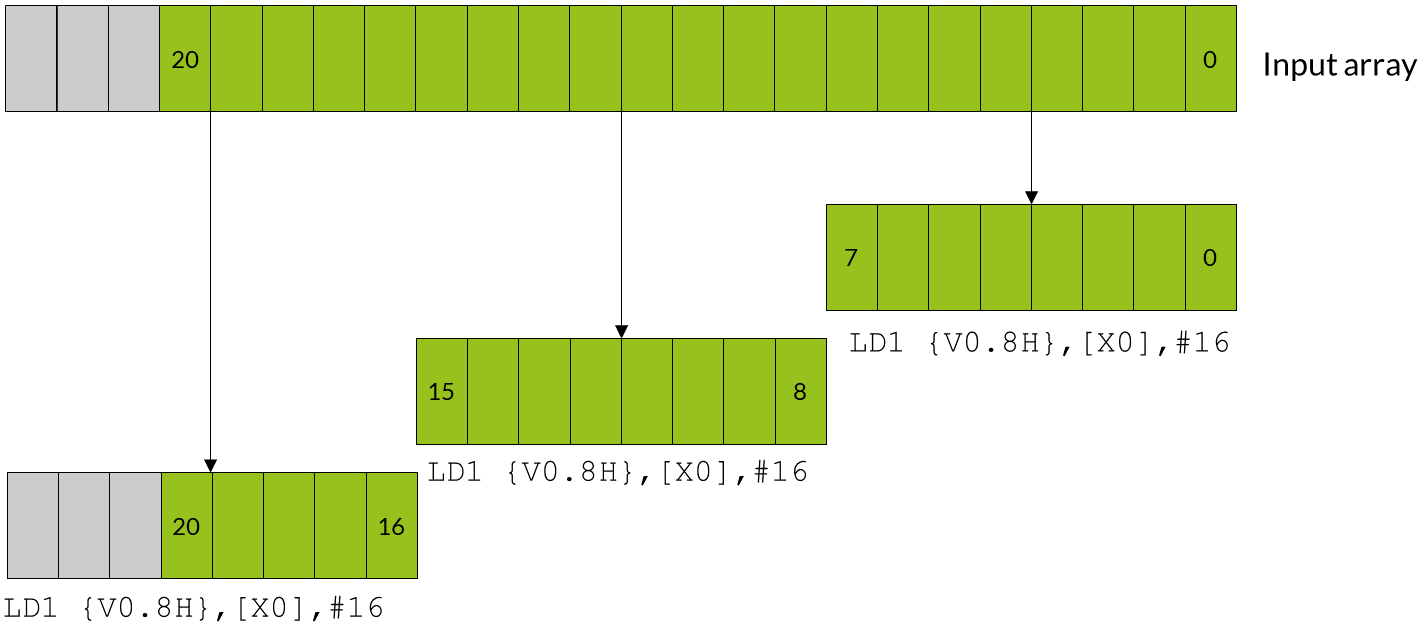The gray data elements in the diagram represent padding values, and the green data elements are the original 21 array values.

Be careful to choose padding values that do not affect the result of your calculation. For example:

• If you are summing array values, use a paddingvalue of zero.
• If you are finding the minimum value in an array, use a padding value of the maximum value that the data element can contain.

It might not be possible to choose a padding value that does not affect the result of your calculation. For example, when calculating the range of an array of numbers any padding value you choose could affect the result. In these cases, do not use this method.

Note:Allocating larger arrays consumes more memory. The increase could be significant if many short arrays are involved.

The following code shows how you could implement a solution that extends arrays with padding:

```// Function entry point
//    X0 = input array pointer
//    X1 = output array pointer
//    X2 = number of elements in array

process_array:
ADD X2, X2, #7    // Add (vector register lanes - 1) to the array length
LSR X2, X2, #3    // Divide the length of the array by the number of
//   vector register lanes (8) to find the number of
//   iterations required.

loop:
LD1 { V0.8H } , [X0], #16  // Load eight elements from the array pointed to
//   by X0 into V0, and update X0 to point to the
//   next vector
//...
//...                      Process the data for this iteration
//...

ST1 { V0.8H } , [X1], #16  // Write eight elements to the output array, and
//   update X1 to point to next vector

SUBS X2, X2, #1            // Decrement the loop counter and set flags
B.NE loop                  // Branch back if count is not yet zero...
RET                        // ... otherwise return```

## Overlap data elements

If the operation is suitable, leftover elements can be handled by overlapping those elements. Overlapping means processing some of the elements in the array twice.

In the example case, the iterations that use overlap would follow these steps:

1. The first iteration processes elements zero to seven.
2. The second iteration processes elements five to 12.
3. The third and final iteration processes elements 13-20.

Note that elements five to seven,  which are the overlap between the first vector and the second vector, are processed twice.

The following diagram shows how all three iterations load eight data elements into the Neon register, with the first and second iterations operating on overlapping vectors: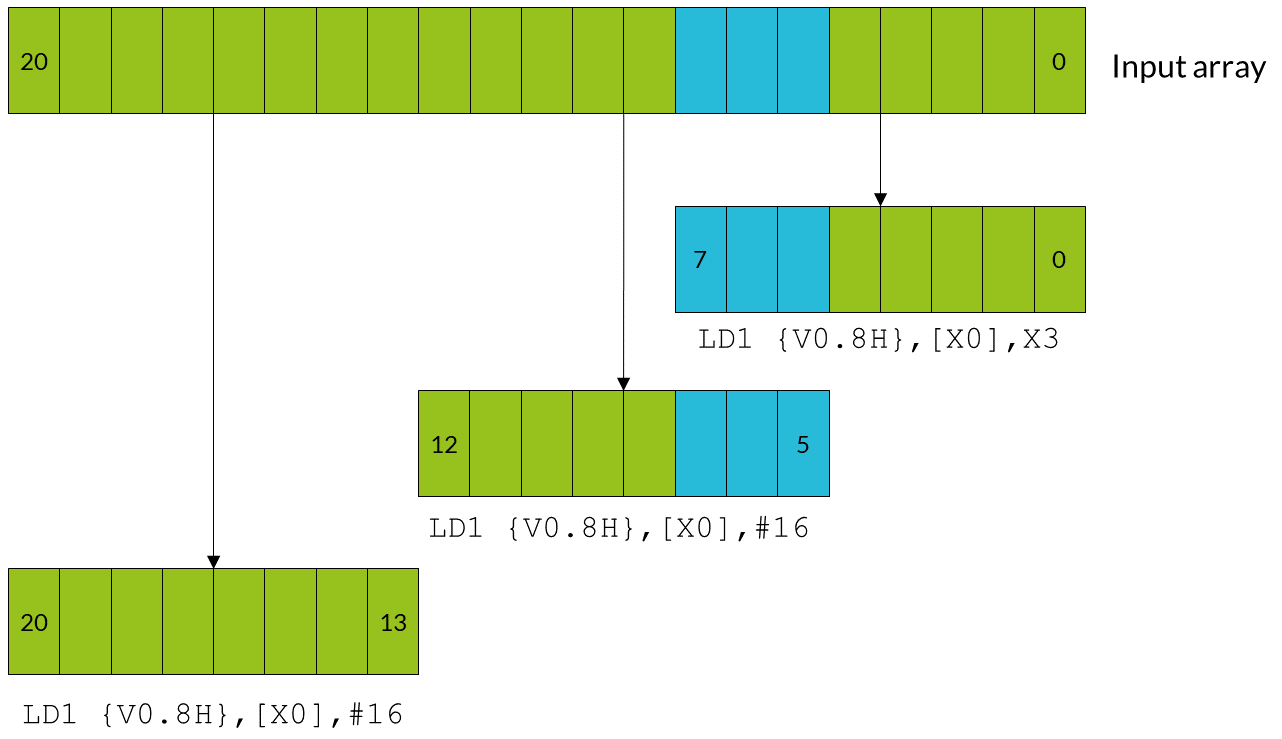The blue data elements represent the overlapping elements that are processed twice. The green data elements are the original 21 array values.

You can only use overlaps when the operation applied to the input data does not vary with the number of times that the operation is applied. The technical term is to say that the operation must be idempotent. For example, if you are trying to find the maximum element in an array, you can use overlaps. This is because it does not matter if the maximum value appears more than once. However, if you are summing an array, you cannot use overlaps. This is because the overlapping elements would be counted twice.

Note:The number of elements in the array must fill at least one complete vector.

The following code shows how you could implement a solution that extends arrays with padding:

```// Function entry point
//    X0 = input array pointer
//    X1 = output array pointer
//    X2 = number of elements in array

process_array:
ANDS X3, X2, #7  // Calculate number of elements left over after
//   processing complete vectors using
//   array length & (vector register lanes - 1).

LSL X3, X3, #1   // Multiply leftover elements by 2 to get the required
//   address increment because we are dealing with doubleword data.

BEQ loopsetup    // If the result of the ANDS is zero, the length
//   of the data is an exact multiple of the number
//   of lanes in the vector register, so there is
//   no overlap. Processing can begin.

// Otherwise, handle the first vector separately...
LD1 {V0.8H}, [X0], X3 //  Load the first eight elements from the array,
//  and update the pointer by the required address increment.
//...
//...                     Process the data for this iteration.
//...

ST1 {V0.8H}, [X1], X3  // Write eight elements to the output array, and
//   update the pointer.

// Now set up the vector processing loop
loopsetup:
LSR  X2, X2, #3      // Divide the length of the array by the number of lanes
//   in the vector register (8) to find the number of
//   iterations required.

//  This loop can now operate on exact multiples
//   of the lane number. The first few elements of
//   the first vector overlap with some of those
//   processed earlier.
loop:
LD1 { V0.8H }, [X0], #16    // Load eight elements from the array pointed to
//   by X0 into V0, and update X0 to point to the
//   next vector.
//...
//...                           Process the data for this iteration.
//...

ST1 { V0.8H }, [X1], #16        // Write eight elements to the output array, and
//   update X1 to point to next vector.

SUBS X2, X2, #1                 // Decrement the loop counter and set flags
B.NE loop                       // Branch back if count is not yet zero...
RET                             // ... otherwise return ```

## Process leftovers as single elements

Neon provides load and store instructions that can operate on single elements in a vector. You can use these instructions to load a partial vector that contains one element, operate on that partial vector, and then write the element back to memory.

In the example case, the iterations using single elements would follow these steps:

1. The first two iterations execute as normal, processing elements zero to seven, and eight to 15.
2. The third iteration needs only to process five elements. A separate loop handles these elements, which loads, processes, and stores single elements.

The following diagram shows how the first two iterations operate on full vectors, while the leftover elements are handled individually: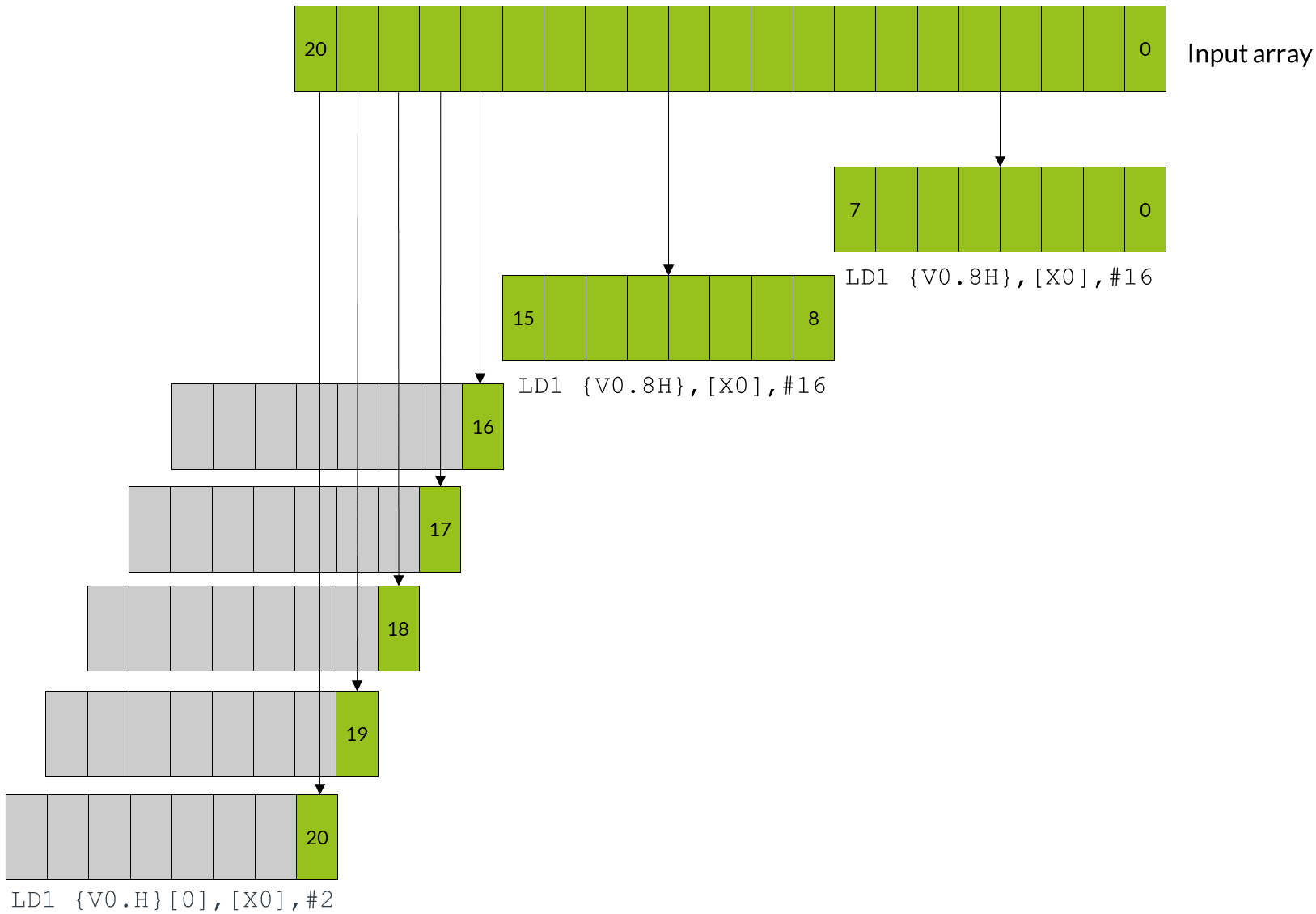This approach is slower than the previous two methods. This is because each leftover element must be loaded, processed, and stored individually.

This approach increases code size. Handling leftovers individually requires two loops, one for the full vectors, and a second loop for the single elements.

Note:Neon single element loads only change the value of the specified lane in the destination element, leaving the rest of the vector intact. If the calculation that you are performing involves instructions that work across a vector, the register must be initialized before loading the first single element. For example, if you were using `ADDV` to sum across the entire vector, initialize the unused lanes to zero.

The following code shows how you could implement a solution that processes leftovers as single elements:

```// Function entry point
//    X0 = input array pointer
//    X1 = output array pointer
//    X2 = number of elements in array

process_array:
LSR X3, X2, #3      // Calculate the number of complete vectors to be
//   processed.

CMP X3, #0
BEQ  singlesetup     // If there are zero complete vectors, branch to
//   the single element handling code.

// Process vector loop.
vectors:
LD1 {V0.8H}, [X0], #16  // Load eight elements from the array and update
//   the pointer by eight doublewords.
//...
//...                   Process the data for this iteration.
//...

ST1 {V0.8H}, [X1], #16  // Write eight elements to the output array, and
//   update the pointer by eight doublewords.
SUBS X3, X3, #1         // Decrement the loop counter, and set flags.

BNE  vectors            // If X3 is not equal to zero, loop.

singlesetup:
ANDS X3, X2, #7         // Calculate the number of single elements to process.
BEQ  exit               // If the number of single elements is zero, branch to exit.

// Process single element loop.
singles:
LD1 {V0.H}, [X0], #2   // Load single element into lane 0, and update the
//   pointer by one doubleword.
//...
//...                     Process the data for this iteration.
//...

ST1 {V0.H}, [X1], #2   // Write the single element in lane zero to the
//   output array, and update the pointer.

SUBS X3, X3, #1           // Decrement the loop counter, and set flags.
BNE  singles              // If X3 is not equal to zero, loop.
exit:
RET```

## Other considerations for leftovers

The three approaches can be refined or adapted to suit your own particular needs as follows:

• Choose when to process leftover elements

You can choose to apply the overlapping and single element techniques at either the start, or the end, of processing an array. The examples in this guide can be adapted to process leftover elements at either end of processing, depending on which is more suitable for your application.

The Armv8-A architecture allows many types of load and store accesses to be arbitrarily aligned.

However, there are exceptions to this rule. For example, load and store addresses should be aligned to cache lines to allow more efficient memory accesses. Check the documentation for your target processor for more information.

• Use A64 base instructions instead of Neon instructions

In the single elements approach, you could use Arm A64 base instructions and the general-purpose registers to operate on each of the single elements, instead of using Neon. However, using both the base A64 instructions and Neon SIMD instructions to write to the same area of memory can reduce performance. The writes from the Arm pipeline are delayed until writes from the Neon pipeline are completed.

Generally, you should avoid writing to the same area of memory, specifically the same cache line, from both Arm and Neon code.

## Permutation - rearranging vectors

When writing programs for SIMD architectures like Neon, performance is often directly related to data ordering. The ordering of data in memory might be inappropriate or suboptimal for the operation that you want to perform.

One solution to these issues might be to rearrange the entire data set in memory before data processing begins. However, this approach is likely to have a high cost to performance. This solution might not even be possible, if your input is a continuous stream of data.

A better solution might be to reorder data values as they are processed. Reordering operations is called permutation. Neon provides a range of permute instructions that typically do the following:

• Take input data from one or more source registers
• Rearrange the data
• Write the result of the permutation to a destination register

## Permutation guidelines

Permutations can help to optimize data processing, but you must remember the following guidelines:

• Permuting data is only useful if it leads to an overall increase in performance for your application. Do you really need to permute your data?
• Permute instructions always have a time cost because they only prepare data. Permute instructions do not process data.
• Different instructions might use different hardware pipelines. An optimal solution maximizes the use of idle pipelines.

When rearranging data, you have the following goals:

• Minimize the number of permute instructions used.
• Choose instructions that are likely to use idle pipelines when they are executed.

## Alternatives to permutation

How can you avoid wasting unnecessary processor cycles on data permutation? Here are some options to consider:

Change the input data structure.

If the input data is well-ordered to begin with, there is no need to rearrange data during loading. However, consider the effects of data locality on cache performance before changing your data structures.

Changing the structure of input data is often not possible, for example when you do not have control over the format.

Another algorithm might be available that better suits the input data.
Modify previous processing stages.
It might be possible to rearrange data more efficiently earlier in the program, especially if the application has a long or complex data pipeline.
Some Neon load and store instructions can interleave and deinterleave data. These interleaving instructions are often used with explicit data permutations, which reduces the total number of instructions required.

You can use any of these approaches, or a combination, to optimize code for Neon.

## Permutation - Neon instructions

Neon provides several different kinds of permute instruction to perform different operations:

## Move instructions

The move instructions copy a sequence of bits into a register. This bit sequence can come either from another register or from a compile-time constant.

The `MOV` instruction has several variants, as shown in the following table:

Instruction Description
`MOV X0, #2` Set X0 to 2.
`MOV X0, X1` Set X0 to the value of X1.
`MOV X0, V3.S`

Set X0 to the value of the second single word (bits 32-63) in V0.

This instruction is an alias of `UMOV`.

`MOV V0, V2.H`

Set every halfword (16 bit) lane in V0, to the value in the third halfword lane of V2.

This instruction is an alias of `DUP`.

`MOV V2.S, S0 `

Set the third single-word lane in V2, to the value of S0.

This instruction is an alias of `INS`.

`MOV s0, v2.S`

Set S0, to the value in the third single-word lane of V2.

This instruction is an alias of `INS`.

The following move instructions specify a sign extension:

Instruction Description
`UMOV X0, V3.S` Set X0 to the zero-extended value of the second single in V3.
`SMOV X0, V3.S` Set X0 to the sign-extended value of the second single in V3.

The following move instructions operate on floating-point values:

The following move instructions specify a sign extension:

Instruction Description
`FMOV S0, #1.0` Set S0, the lowest 32 bits of V0, to the floating-point value 1.0.
`FMOV V0.8H, #2.0` Set all eight halfword (16-bit) lanes in V0 to the floating-point value 2.0.
`FMOV D1, D4` Set D1 to the value of D4.

All these move instructions have the following in common:

• The instructions copy a single fixed sequence of bits into one or more lanes in a destination register.
• The instructions do not perform any floating-point type conversion.

If you need to move more than one value, see the other instructions below. Floating point conversions are beyond the scope of this guide.

## Reverse instructions

The reverse instructions break a vector into ordered containers. The ordering of these containers is preserved. These containers are then split into ordered subcontainers. Within each container, the ordering of subcontainers is reversed. The newly ordered elements are then copied into the destination register.

For example, consider the following instruction:

`REV16 v0.16B, v1.16B`

This instruction splits the 128-bit V1 register into eight 16-bit halfword containers. Each of these halfword containers is then split into a pair of one-byte subcontainers. Each pair of subcontainers is then reversed, as shown in the following diagram: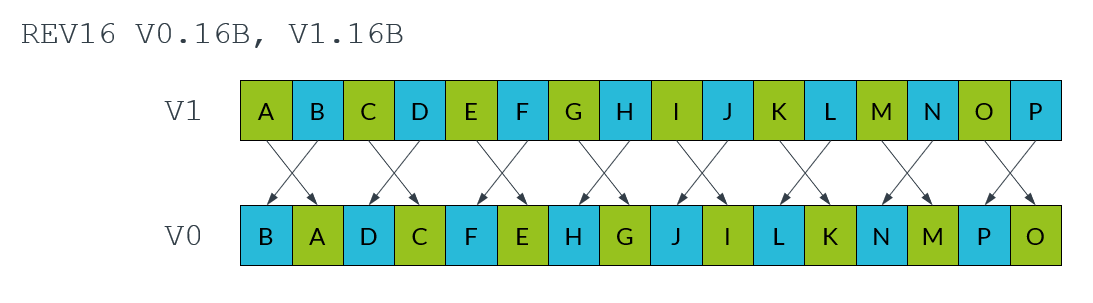There are several reverse instructions to handle different sizes of containers and subcontainers, as shown in the following tables and diagrams:

Instruction Number of containers Size of containers Number of subcontainers in each container Size of subcontainers
`REV16 v0.16B, v1.16B` 8 16-bit 2 8-bit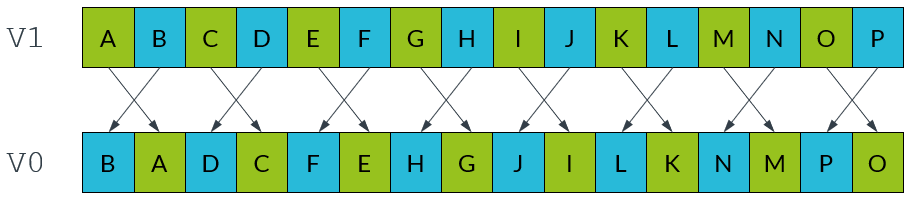Instruction Number of containers Size of containers Number of subcontainers in each container Size of subcontainers
`REV32 v0.16B, v1.16B ` 4 32-bit 4 8-bit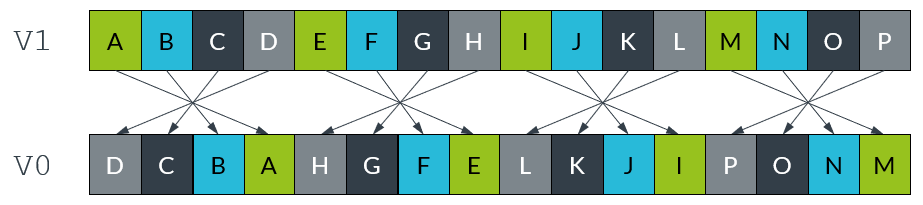Instruction Number of containers Size of containers Number of subcontainers in each container Size of subcontainers
`REV32 v0.8H, v1.8H` 4 32-bit 2 16-bit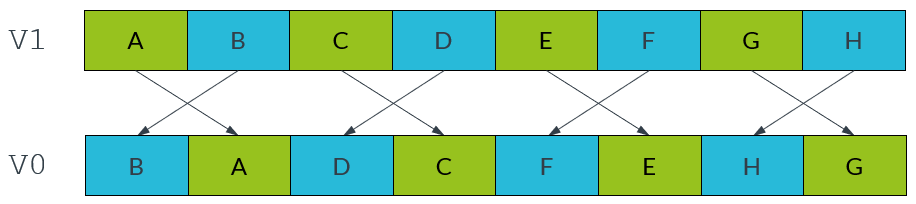Instruction Number of containers Size of containers Number of subcontainers in each container Size of subcontainers
`REV64 v0.16B, v1.16B` 2 64-bit 8 8-bit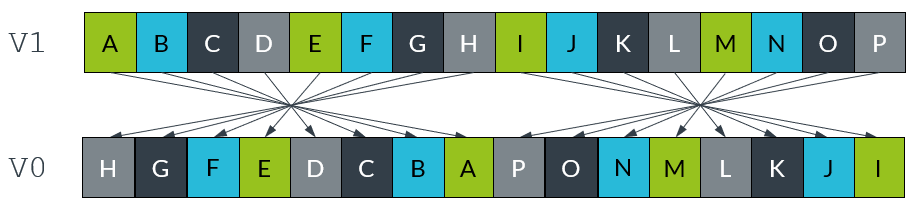Instruction Number of containers Size of containers Number of subcontainers in each container Size of subcontainers
`REV64 v0.8H, v1.8H` 2 64-bit 2 16-bit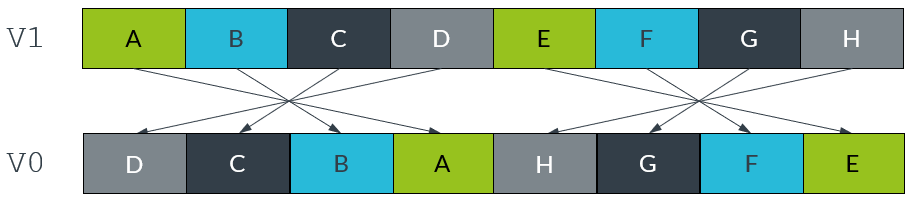Instruction Number of containers Size of containers Number of subcontainers in each container Size of subcontainers
`REV64 v0.4S, v1.4S` 2 64-bit 2 32-bit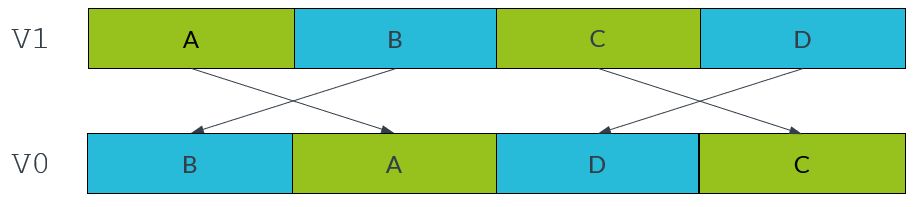## Extraction instructions

The extract instruction, `EXT`, creates a new vector by extracting consecutive lanes from two different source vectors. An index number, n, specifies the lowest lane from the first source vector to include in the destination vector. This instruction lets you create a new vector that contains elements that straddle a pair of existing vectors.

The `EXT` instruction constructs the new vector by doing the following:

1. From the first source vector, copy the lower n lanes to the highest lanes in the destination vector.
2. From the second source vector, ignore the lower n lanes and copy the remaining lanes to the lowermost lanes in the destination vector.

For example, the following instruction uses an index with value 3:

`EXT v0.16B, v1.16B, v2.16B, #3`

This instruction extracts lanes as follows:

1. Copy the lowest 3 bytes from V1 into the highest 3 bytes of V0.
2. Copy the highest 13 bytes of V2 into the lowest 13 bytes of V1.

The following diagram illustrates the extraction process: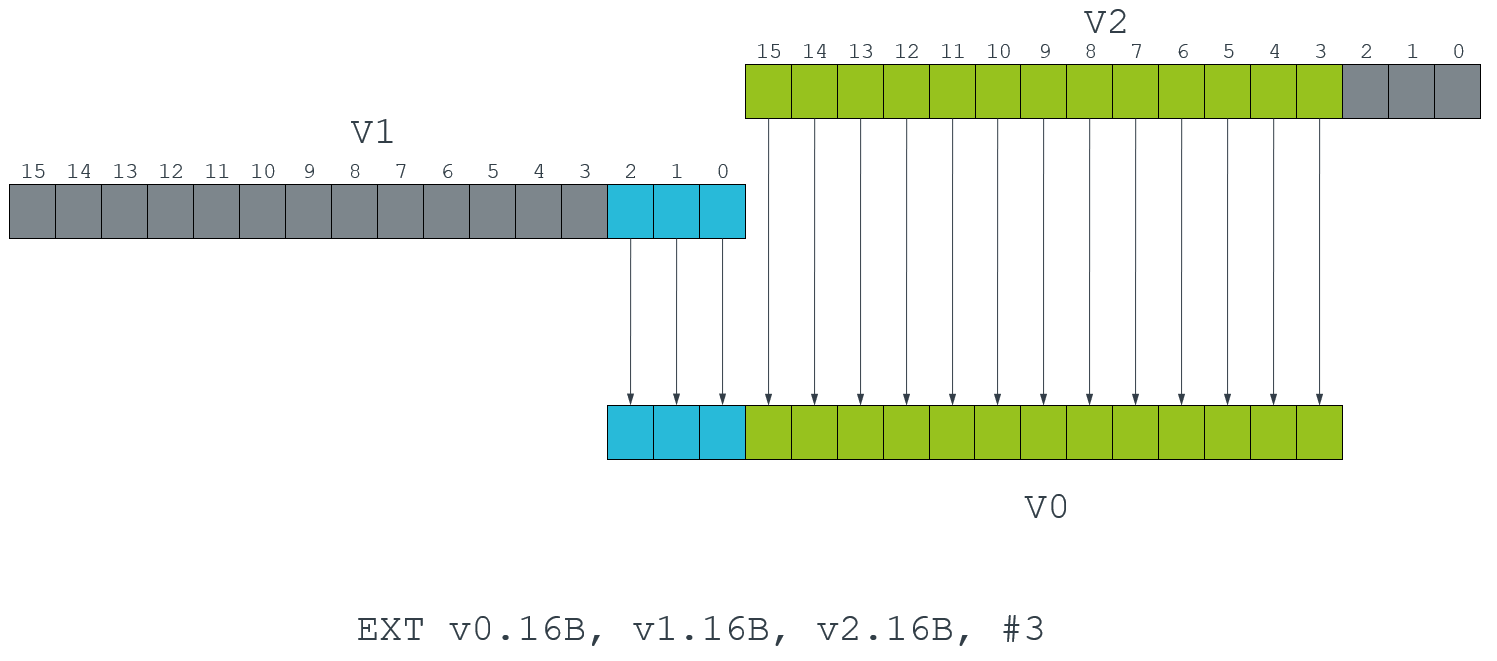The other extraction instructions are less general. They copy all the values from a source register, then place them into smaller lanes in the destination, as follows:

• `XTN` Extract and narrow

Reads each vector element from the source register, narrows each value to half the original width, and writes the resulting vector to the lower half of the destination register. The upper half of the destination register is cleared.

The following diagram shows the operation of the `XTN` instruction: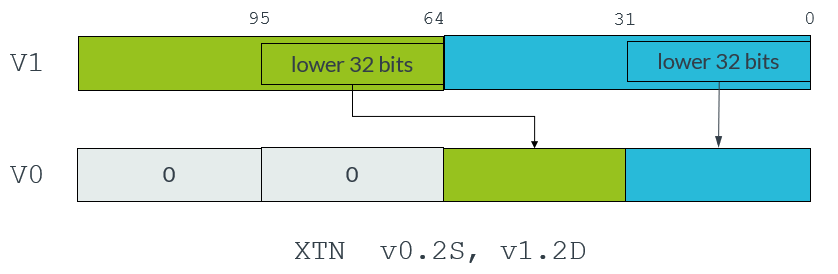• `XTN2` Extract and narrow into upper halves

Reads each vector element from the source register, narrows each value to half the original width, and writes the resulting vector to the upper half of the destination register. The other bits of the destination register are not affected.

The following diagram shows the operation of the `XTN2` instruction: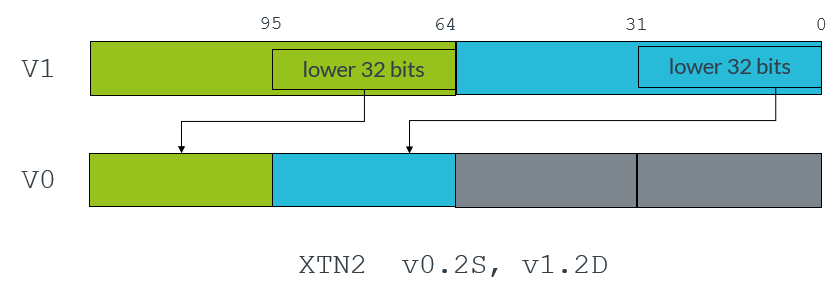With both the `XTN` and `XTN2` instructions, the destination vector elements are half as long as the source vector elements.

Neon provides several variants of the extraction instructions for different combinations of sign and overflow behavior. The following table shows these extraction instruction variants:

Table 1‑1

 Instruction Description `SQXTN` Signed saturating extract and narrow. All values are signed integer values. Large values saturate to the maximum positive or negative integer value. `SQXTN2` Signed saturating extract and narrow into upper halves. All values are signed integer values. Large values saturate to the maximum positive or negative integer value. `SQXTUN` Signed saturating extract and unsigned narrow. Source values are signed, destination values are unsigned. Large values saturate to the maximum positive integer value or zero. Other values are zero extended. `SQXTUN2` Signed saturating extract and unsigned narrow into upper halves. Source values are signed, destination values are unsigned. Large values saturate to the maximum positive integer value or zero. Other values are zero extended. `UQXTN` Unsigned saturating extract and narrow. All values are unsigned integer values. Large values saturate to the maximum positive integer value or zero. Other values are zero extended. `UQXTN2` Unsigned saturating extract and narrow into upper halves. All values are unsigned integer values. Large values saturate to the maximum positive integer value or zero. Other values are zero extended.

## Transpose instructions

The transpose instructions interleave elements from two source vectors. Neon provides two transpose instructions: `TRN1` and `TRN2`.

`TRN1` interleaves the odd-numbered lanes from the two source vectors, while `TRN2` extracts the even-numbered lanes. The following diagram shows this process: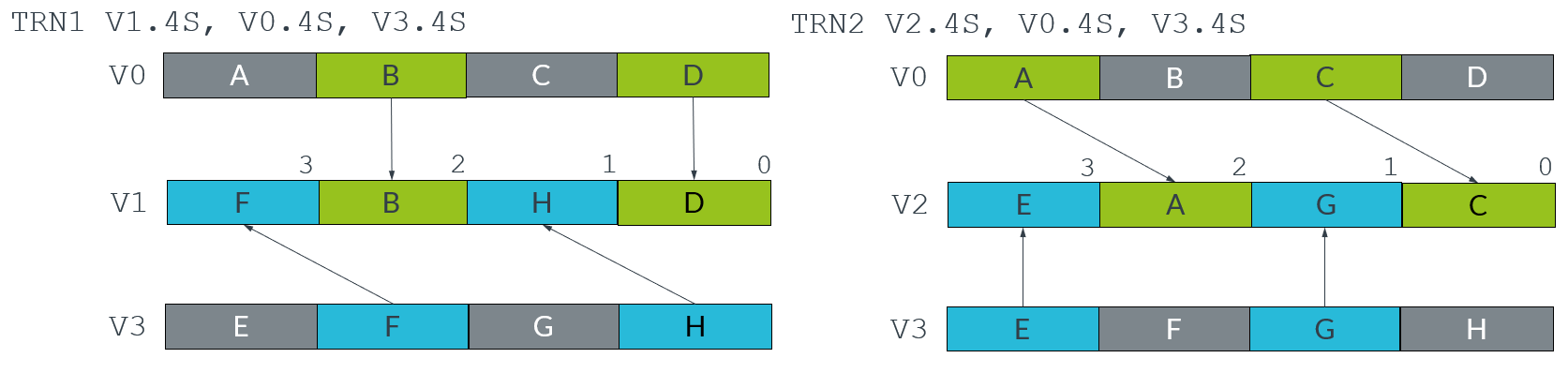In mathematics, the transpose of a matrix is an operation that switches the rows and columns. For example, the following diagram shows the transpose of a 2x2 matrix: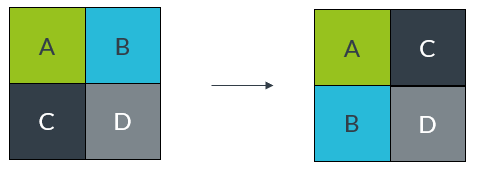We can use the Neon transpose instructions to transpose matrices.

For example, consider the following two matrices: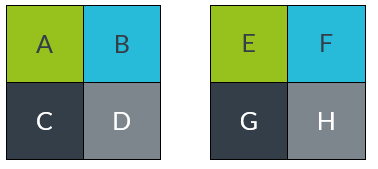We can store these matrices across two Neon registers, with the top row in V0 and the bottom row in V1, as shown in the following diagram: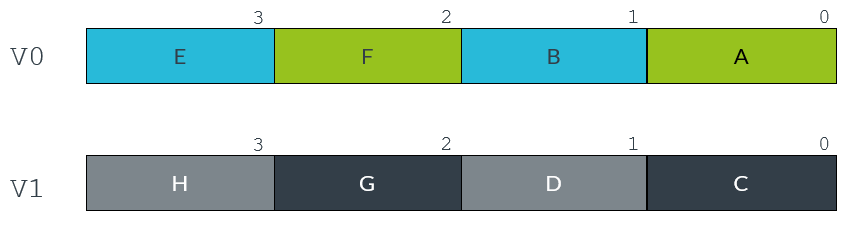The following instructions transpose this matrix into the destination registers V2 and V3:

```TRN1 v2.4s, v0.4S, v1.4S
TRN2 v3.4s, v0.4S, v1.4S```

The following diagram illustrates this process: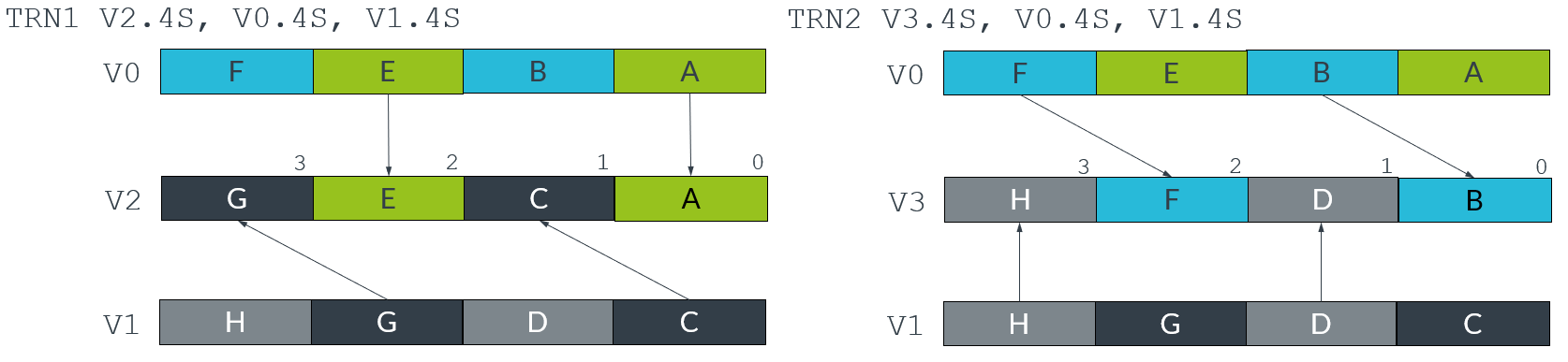The following diagram shows the transposed matrices: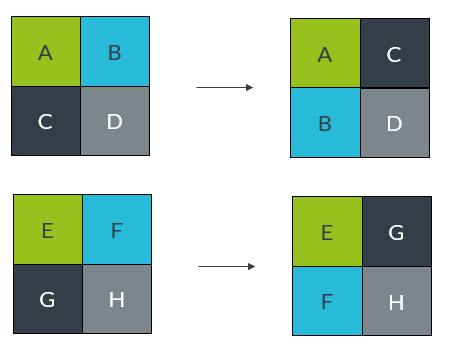## Interleave instructions

Like the transpose instructions, the zip instructions use interleaving to form vectors. `ZIP1` takes the lower halves of two source vectors, and fills a destination vector by interleaving the elements in those two lower halves. `ZIP2` does the same thing with the upper halves of the source vectors.

For example, the following instructions create an interleaved vector that is stored across two registers, V1 and V2:

```ZIP1 V2.16B, V4.16B, V3.16B
ZIP2 V1.16B, V4.16B, V3.16B```

This result vector is formed by alternating elements from the two source registers, V1 and V2. The `ZIP1` instruction creates the lower half of the result vector in V2, and the `ZIP2` instruction creates the upper half in V1. The following diagram shows this process: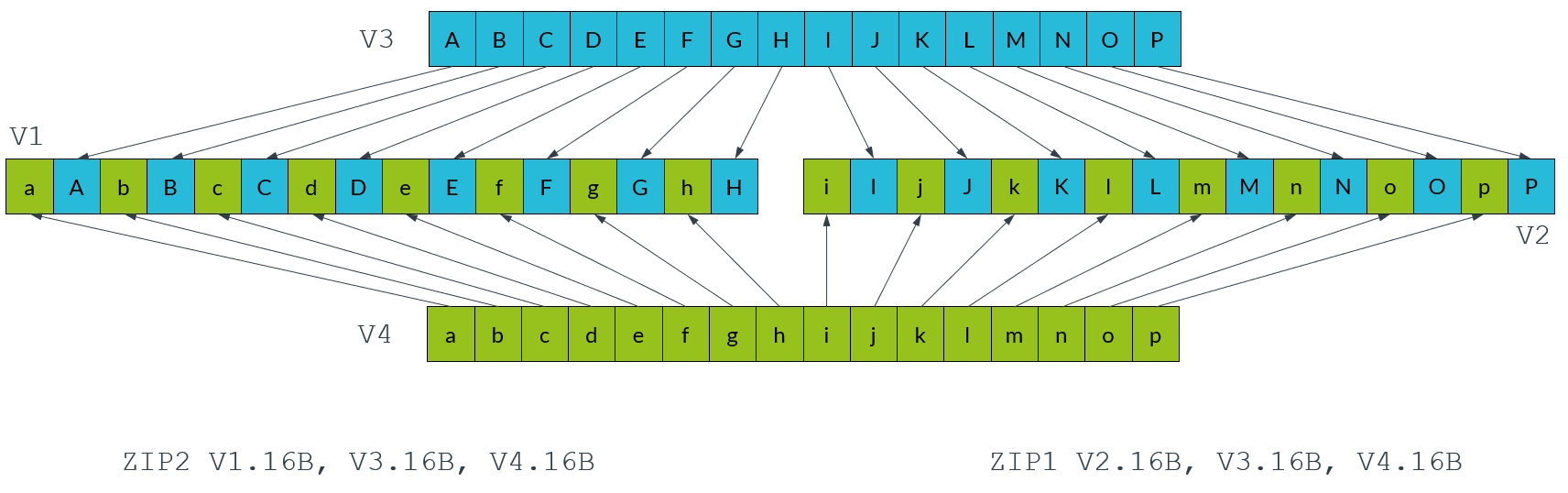The `UZIP1` and `UZIP2` instructions perform the reverse operation, deinterleaving alternate elements into two separate vectors.

## Table lookup instructions

All the permute instructions that we have described have one thing in common: the pattern of the permutation is fixed. To perform arbitrary permutations, Neon provides the table lookup instructions `TBL` and `TBX`.

The `TBL` and `TBX` instructions take two inputs:

• An index input, consisting of one vector register containing a series of lookup values
• A lookup table, consisting of a group of up to four vector registers containing data

The instruction reads each lookup value from the index, and uses that lookup value to retrieve the corresponding value from the lookup table.

For example, the following instruction provides a vector of lookup values in V0, and a lookup table consisting of two registers: V1 and V2:

`TBL V3.8D, {v1.16B, v2.16B}, v2.4S`

The value in lane 0 of V0 is 6, so the value from lane 6 of V1 is copied into the first lane of the destination register V4. The process continues for all the other lookup values in V0, as shown in the following diagram: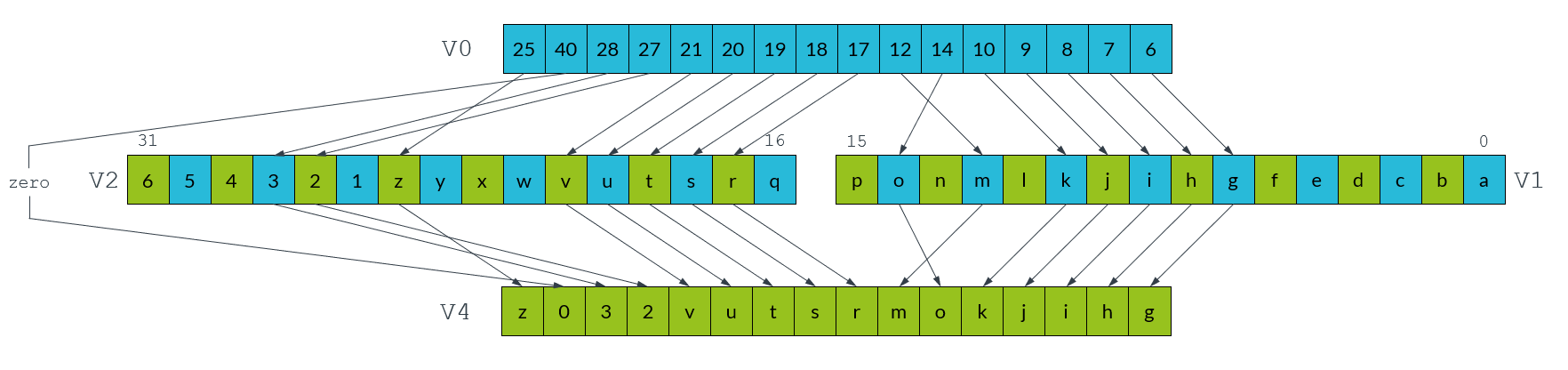The `TBL` and `TBX` instructions only differ in how they handle out of range indices. `TBL` writes a zero if an index is out-of-range, while `TBX` leaves the original value unchanged in the destination register. In the above example, lane 14 in V0 contains the lookup value, 40. Because the lookup table only contains two registers, the range of indices is 0-31. Lane 14 in the destination vector is therefore set to zero.

The `TBL` and `TBX` instructions are very powerful, so only use these instructions when necessary. On most systems a short sequence of fixed pattern permutations is faster.

## Matrix multiplication

In this section of the guide, we look at how you can use Neon to perform an example data processing task. Specifically, we show you how to efficiently multiply four-by-four matrices together, an operation frequently used in the world of 3D graphics. We assume that the matrices are stored in column-major order because OpenGL ES uses this format.

Note: Download the code for the functions that are described in this section here: matrix_asm_a64.s.zip

### The algorithm

First, we will look at the algorithm that multiplies two matrices together. We expand the calculation to examine the matrix multiplication operation in detail, then identify operations that we can implement using Neon instructions.

The following diagram shows how to calculate the first column of results when multiplying two matrices together: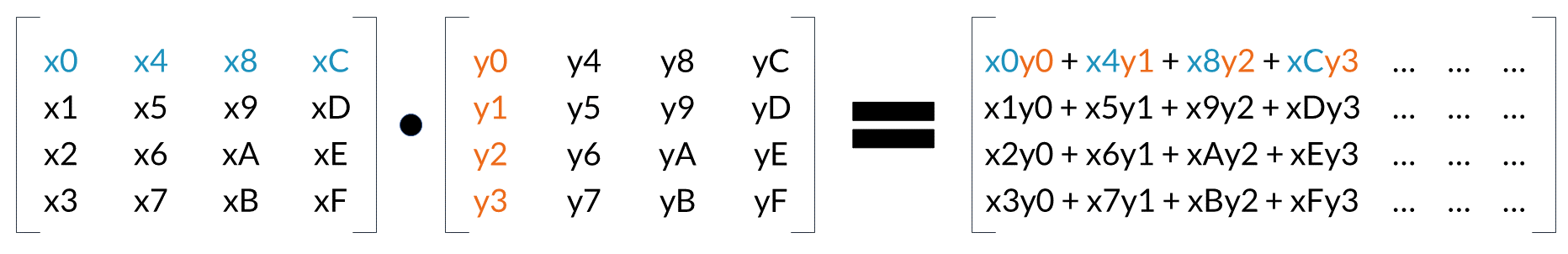Look at the first element in the result matrix. Every element in the first row of the first matrix (blue) is multiplied by the corresponding element in the first column of the second matrix (orange). We accumulate the results to give the first result value. This process is repeated for all the remaining elements in the result matrix.

The following diagram shows how we can use the Neon FMUL vector-by-scalar multiplication instruction to calculate these results: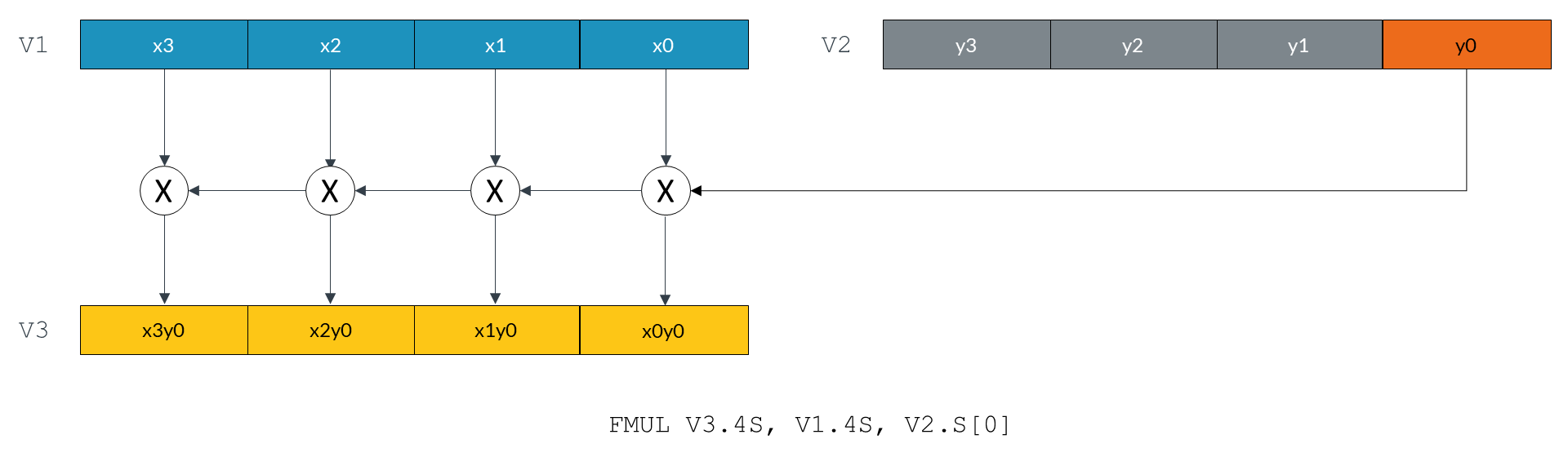The `FMUL` instruction in the preceding diagram multiplies every element of the vector in the V1 register by the scalar value in lane 0 of the V2 register. The instruction then stores the resulting vector in the V3 register.

The following diagram shows how this single instruction calculates the first term for each of the values in the first column of the result matrix: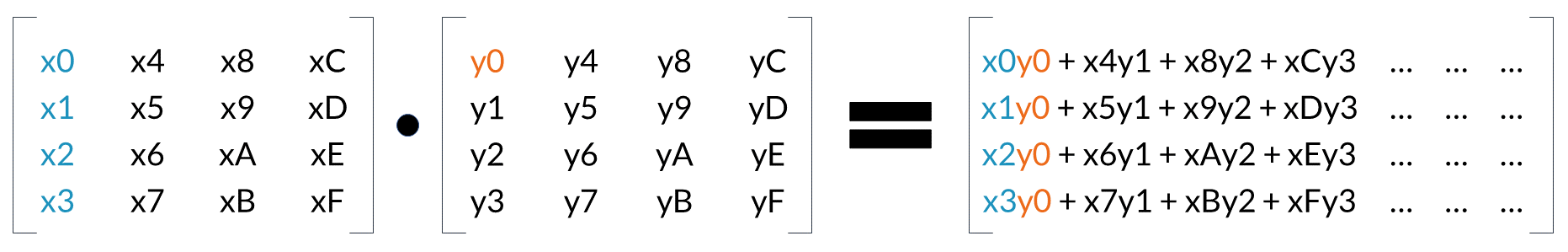We can use the same method to calculate the remaining terms. However, this time we will use the `FMLA` multiply and accumulate instruction to sum the terms.

Because we are operating on the columns of the first matrix and producing a column of results, reading and writing elements is a linear operation. Interleaving load or store instructions are not required.

### Neon registers and data size

The Neon register file is a collection of registers that can be accessed as either 64-bit or 128-bit registers.

The number of lanes in a Neon vector depends on the size of the vector and the data elements in the vector. The following diagram shows the different ways that you can arrange and access data in Neon registers: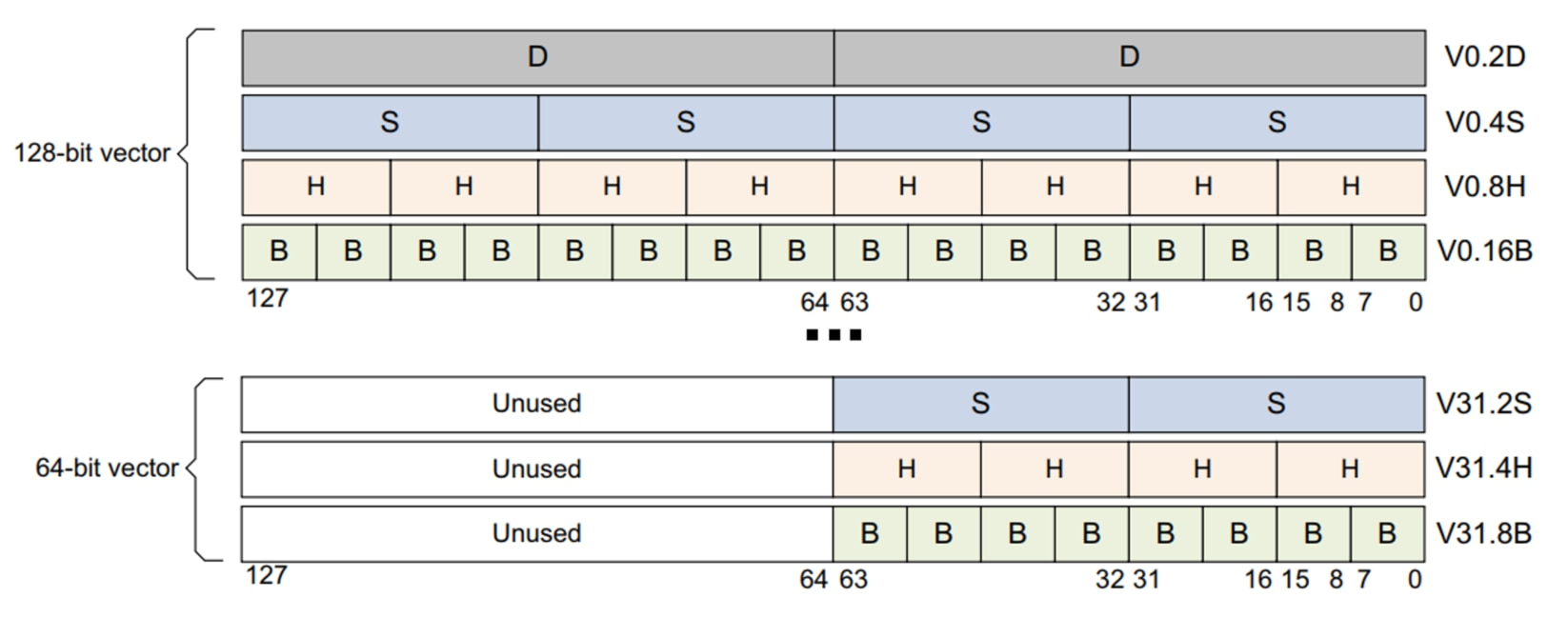This guide examines two different implementations of the matrix multiplication algorithm. Each implementation performs multiplication in a different way:

• The floating-point implementation operates on values using the 32-bit floating-point format.

Multiplying two 32-bit floating-point numbers gives a result that is another 32-bit number. This means that the floating-point implementation uses the 4S vector lane format throughout.

• The fixed-point implementation operates on values using the 16-bit Q1.14 fixed-point format.

Multiplying two 16-bit Q1.14 fixed-point format numbers together gives a 32-bit result that must be narrowed to 16 bits. This means that we can use the 4H vector lane format for the 16-bit input and result values, but the 4S vector lane format for the intermediate multiplication result.

### Floating-point implementation

The floating-point implementation multiplies two matrices that contain 32-bit floating-point numbers.

The implementation has three stages:

1. Load the matrix data from memory to Neon registers.
2. Perform the matrix multiplication operation.
3. Store the result matrix back to memory.

The following code shows how we load the data into the Neon registers:

```LD1  {V0.4S, V1.4S, V2.4S, V3.4S}, [X1]
LD1  {V4.4S, V5.4S, V6.4S, V7.4S}, [X2] ```

Our matrices are stored in column-major order. This means that the column data is stored linearly in memory. We use the `LD1` instruction to load data from memory into the Neon registers V0 - V7.

Neon provides 32 registers. Each register is 128 bits wide. We can load all the elements from both input matrices into registers, and still have registers left over to use as accumulators. In this implementation, registers V0-V3 hold the 16 elements from the first matrix, and registers V4-V7 hold the 16 elements from the second matrix. Each 128-bit register holds four 32-bit values, representing an entire matrix column.

Similarly, the following code shows how we use the `ST1` instruction to store the result back to memory:

`ST1  {V8.4S, V9.4S, V10.4S, V11.4S}, [X0]`

The following code shows how we calculate a column of results using just four Neon multiply instructions:

```FMUL V8.4S, V0.4S, V4.S           // rslt col0  = (mat0 col0) * (mat1 col0 elt0)
FMLA V8.4S, V1.4S, V4.S           // rslt col0 += (mat0 col1) * (mat1 col0 elt1)
FMLA V8.4S, V2.4S, V4.S           // rslt col0 += (mat0 col2) * (mat1 col0 elt2)
FMLA V8.4S, V3.4S, V4.S           // rslt col0 += (mat0 col3) * (mat1 col0 elt3)```

The first `FMUL` instruction implements the operation that is highlighted in the previous diagram. Matrix elements x0, x1, x2, and x3 (in the four lanes of register V0) are each multiplied by y0 (element 0 in register V4), and the result stored in V8.

Subsequent `FMLA` instructions operate on the other columns of the first matrix, multiplying by corresponding elements of the first column of the second matrix. Results are accumulated into V8 to give the first column of values for the result matrix.

If we only need to calculate a matrix-by-vector multiplication, the operation is now complete. However, to complete the matrix-by-matrix multiplication, we must execute three more iterations. These iterations use values y4 to yF in registers V5 toV7.

The following code shows the full implementation of a four-by-four floating-point matrix multiply:

```matrix_mul_float:
LD1  {V0.4S, V1.4S, V2.4S, V3.4S}, [X1] // load all 16 elements of matrix 0 into
//  V0-V3, four elements per register
LD1  {V4.4S, V5.4S, V6.4S, V7.4S}, [X2] // load all 16 elements of matrix 1 into
//  V4-V7, four elements per register

FMUL V8.4S, V0.4S, V4.S     // rslt col0  = (mat0 col0) * (mat1 col0 elt0)
FMUL V9.4S, V0.4S, V5.S     // rslt col1  = (mat0 col0) * (mat1 col1 elt0)
FMUL V10.4S, V0.4S, V6.S    // rslt col2  = (mat0 col0) * (mat1 col2 elt0)
FMUL V11.4S, V0.4S, V7.S    // rslt col3  = (mat0 col0) * (mat1 col3 elt0)

FMLA V8.4S, V1.4S, V4.S     // rslt col0 += (mat0 col1) * (mat1 col0 elt1)
FMLA V9.4S, V1.4S, V5.S     // rslt col1 += (mat0 col1) * (mat1 col1 elt1)
FMLA V10.4S, V1.4S, V6.S    // rslt col2 += (mat0 col1) * (mat1 col2 elt1)
FMLA V11.4S, V1.4S, V7.S    // rslt col3 += (mat0 col1) * (mat1 col3 elt1)

FMLA V8.4S, V2.4S,  V4.S    // rslt col0 += (mat0 col2) * (mat1 col0 elt2)
FMLA V9.4S, V2.4S,  V5.S    // rslt col1 += (mat0 col2) * (mat1 col1 elt2)
FMLA V10.4S, V2.4S, V6.S    // rslt col2 += (mat0 col2) * (mat1 col2 elt2)
FMLA V11.4S, V2.4S, V7.S    // rslt col3 += (mat0 col2) * (mat1 col2 elt2)

FMLA V8.4S, V3.4S, V4.S     // rslt col0 += (mat0 col3) * (mat1 col0 elt3)
FMLA V9.4S, V3.4S, V5.S     // rslt col1 += (mat0 col3) * (mat1 col1 elt3)
FMLA V10.4S, V3.4S, V6.S    // rslt col2 += (mat0 col3) * (mat1 col2 elt3)
FMLA V11.4S, V3.4S, V7.S    // rslt col3 += (mat0 col3) * (mat1 col3 elt3)

ST1  {V8.4S, V9.4S, V10.4S, V11.4S}, [X0] // store all 16 elements of result

### Fixed-point implementation

Using fixed-point arithmetic for calculations is often faster than using floating-point arithmetic. Fixed-point arithmetic requires less memory bandwidth than floating-point arithmetic to read and write values that use fewer bits. Because fixed-point arithmetic uses integer data types, multiplication of fixed-point values is usually quicker than the same operations applied to floating point numbers.

However, when using fixed-point arithmetic, you must choose the representation carefully, so that you can avoid overflow or saturation. At the same time, you must preserve the degree of precision in the results that your application requires.

Implementing a matrix multiply using fixed-point values is very similar to floating-point. This example uses Q1.14 fixed-point format, but the operations are similar for other formats. Adapting this example to another fixed-point format might only require a change to the final shift that is applied to the accumulator.

Our fixed-point implementation uses a macro to perform the matrix multiplication, as shown in the following code:

```  .macro mul_col_s16 res_d, col_d
SMULL V12.4S, V0.4H, \col_d\().H // multiply col element 0 by matrix col 0
SMLAL V12.4S, V1.4H, \col_d\().H // multiply col element 0 by matrix col 1
SMLAL V12.4S, V2.4H, \col_d\().H // multiply col element 0 by matrix col 2
SMLAL V12.4S, V3.4H, \col_d\().H // multiply col element 0 by matrix col 3
SQSHRN \res_d\().4H, V12.4S, #14    // shift right and narrow accumulator into
//  Q1.14 fixed-point format, with saturation
.endm```

Comparing the fixed-point implementation to the floating-point implementation, the major differences are:

• Matrix values are now 16-bit instead of 32-bit. Because of this difference, we use the 4H configuration to store four 16-bit values in the lower 64 bits of the 128-bit Neon register.
• The result of multiplying two 16-bit numbers is a 32-bit number. We use the signed multiply long `SMULL` and signed multiply-add long `SMLAL` instructions to store the results in the 32-bit 4S lane configuration of the Neon register.
• The final result matrix must contain 16-bit values, but the accumulators contain 32-bit values. We obtain a 16-bit result using the `SQSHRN` signed saturating shift right narrow instruction. This instruction adds the correct rounding value to each element, shifts it right, and saturates the result to the new, narrower element size.

The following code shows the full implementation of a four-by-four fixed-point matrix multiply:

```  .macro mul_col_s16 res_d, col_d
SMULL V12.4S, V0.4H, \col_d\().H   // multiply col element 0 by matrix col 0
SMLAL V12.4S, V1.4H, \col_d\().H   // multiply col element 0 by matrix col 1
SMLAL V12.4S, V2.4H, \col_d\().H   // multiply col element 0 by matrix col 2
SMLAL V12.4S, V3.4H, \col_d\().H   // multiply col element 0 by matrix col 3
SQSHRN \res_d\().4H, V12.4S, #14      // shift right and narrow accumulator into
//  Q1.14 fixed-point format, with saturation
.endm

.global matrix_mul_fixed
matrix_mul_fixed:
LD1  {V0.4H, V1.4H, V2.4H, V3.4H}, [X1] // load all 16 elements of matrix 0
// into V0-V3, four elements per register
LD1  {V4.4H, V5.4H, V6.4H, V7.4H}, [X2] // load all 16 elements of matrix 1
// into V4-V7, four elements per register

mul_col_s16 v8, v4                        // matrix 0 * matrix 1 col 0
mul_col_s16 v9, v5                        // matrix 0 * matrix 1 col 1
mul_col_s16 v10, v6                       // matrix 0 * matrix 1 col 2
mul_col_s16 v11, v7                       // matrix 0 * matrix 1 col 3

ST1  {V8.4H, V9.4H, V10.4H, V11.4H}, [X0] // store all 16 elements of result

### Optimized instruction scheduling

The fixed-point implementation uses a macro to perform the main multiplication operation on each matrix column. In the macro, adjacent multiply instructions write to the same register: V12. This means that each Neon pipeline must wait for each multiply to complete before it can start the next instruction. The following code repeats the macro from the fixed-point implementation:

```.macro mul_col_s16 res_d, col_d
SMULL V12.4S, V0.4H, \col_d\().H // multiply col element 0 by matrix col 0
SMLAL V12.4S, V1.4H, \col_d\().H // multiply col element 0 by matrix col 1
SMLAL V12.4S, V2.4H, \col_d\().H // multiply col element 0 by matrix col 2
SMLAL V12.4S, V3.4H, \col_d\().H // multiply col element 0 by matrix col 3
SQSHRN \res_d\().4H, V12.4S, #14    // shift right and narrow accumulator into
//  Q1.14 fixed-point format, with saturation
.endm```

If we take the instructions out of the macro and rearrange them, we can separate instructions that write to the same register. This reduces the risk of register contention and allows instructions to make efficient use of the Neon pipeline.

The following code shows how to rearrange and optimize accesses to the accumulator registers:

```SMULL V12.4S, V0.4H, V4.H
SMULL V13.4S, V0.4H, V5.H
SMULL V14.4S, V0.4H, V6.H
SMULL V15.4S, V0.4H, V7.H
SMLAL V12.4S, V1.4H, V4.H
SMLAL V13.4S, V1.4H, V5.H
SMLAL V14.4S, V1.4H, V6.H
SMLAL V15.4S, V1.4H, V7.H

SMLAL V12.4S, V2.4H, V4.H
SMLAL V13.4S, V2.4H, V5.H
SMLAL V14.4S, V2.4H, V6.H
SMLAL V15.4S, V2.4H, V7.H

SMLAL V12.4S, V3.4H, V4.H
SMLAL V13.4S, V3.4H, V5.H
SMLAL V14.4S, V3.4H, V6.H
SMLAL V15.4S, V3.4H, V7.H

SQSHRN V8.4H, V12.4S, #14
SQSHRN V9.4H, V13.4S, #14
SQSHRN V10.4H, V14.4S, #14
SQSHRN V11.4H, V15.4S, #14   ```

## Shifting left and right

This section of the guide introduces the different shift operations that are provided by Neon. An example shows how to use these shifting operations to convert image data between commonly used color depths.

### Shifting vectors

Neon vector shifts are very similar to shifts in scalar Arm code. A shift moves the bits in each element of a vector left or right. Bits that fall off the left or right of each element are discarded. These discarded bits are not shifted to adjacent elements.

The number of bits to shift can be specified as follows:

• With a single immediate literal encoded in the instruction
• With a shift vector

When using a shift vector, the shift that is applied to each element of the input vector depends on the corresponding element in the shift vector. The elements in the shift vector are signed values. This means that left, right, and zero shifts are possible, on a per-element basis. The following diagram shows an input vector, v0, and a shift vector v1: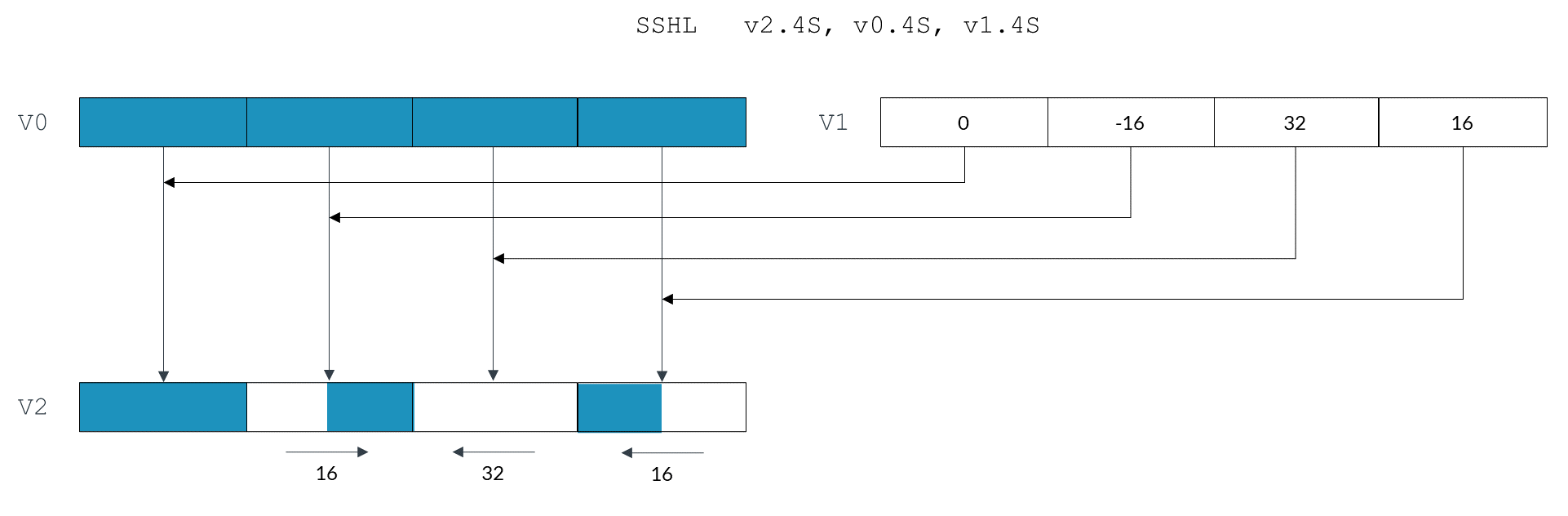Each vector element shifts as follows:

• Element 0, in the right-most lane of v0, shifts left by 16 bits.
• Element 1 of v0 shifts left by 32 bits. Because the width of the element is also 32 bits, the final value of this element is zero.
• Element 2 of v0 shifts right by 16 bits. The negative value in v1 changes the left shift to a right shift.
• Element 3, in the left-most lane of v0, is unchanged. This is because the zero value in v1 means no shift.

The negative shift value -16 corresponding to element 2 changes the left shift operation to a right shift. When shifting right, we must consider whether we are dealing with signed or unsigned data. Because the `SSHL` instruction is a signed shift operation, the new 16 bits introduced in the top half of this element are the same as the top bit of the original element value. That is, the signed shift `SSHL` is a sign-extending shift. If we use the unsigned `USHL` instruction instead of the signed `SSHL` instruction, the new 16 bits would all be zeroes.

### Shifting and inserting

Neon also supports shifts with insertion. This operation lets you combine bits from two vectors. For example, the `SLI` shift left and insert instruction shifts each element of the source vector left. The new bits that are inserted at the right of each element are the corresponding bits from the destination vector.

The following image shows two vector registers v1 and v2, each containing four elements. The `SLI` instruction takes each element from v1, shifts it left by 16 bits, then combines it with the corresponding element in v0.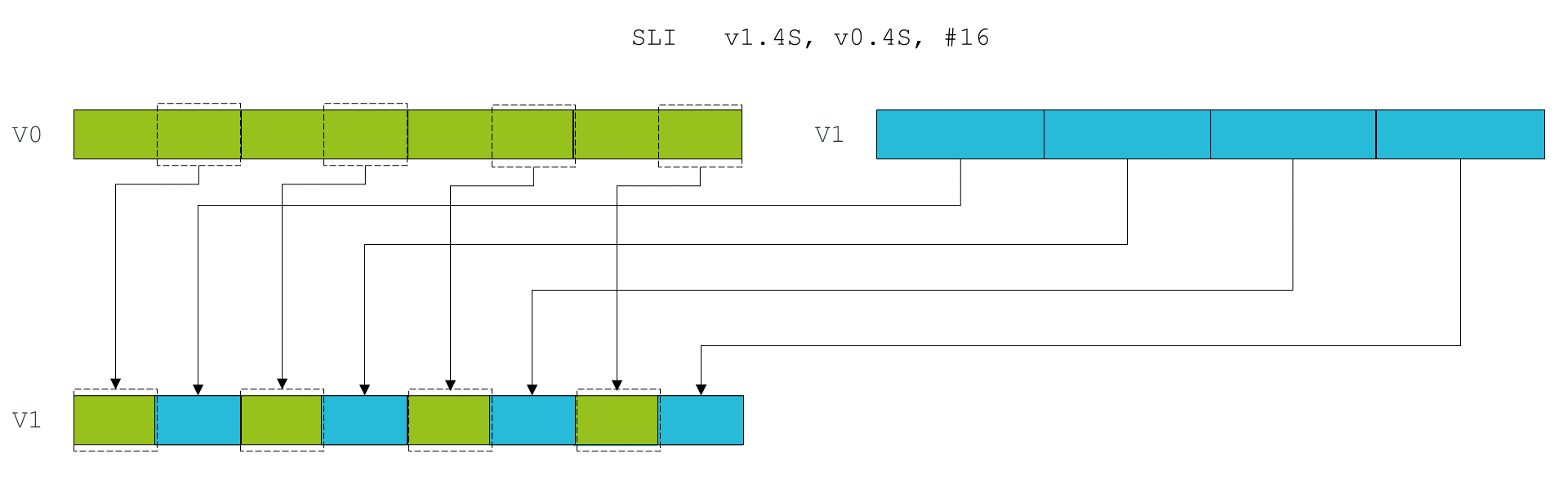### Shifting and accumulation

Finally, the Neon instruction `SSRA` supports shifting the elements of a vector right, and accumulating the results into another vector. This instruction is useful for situations in which interim calculations are made at a high precision, before the result is combined with a lower precision accumulator.

### Instruction modifiers

Each shift instruction can take one or more modifiers. These modifiers do not change the shift operation itself, however the inputs or outputs are adjusted to remove bias or saturate to a range.

The general format of shift instructions with modifiers are as follows:

`[<sign>[<sat>]][<round>]SH<dir>[<scale>]`

Where the modifiers are as follows:

Modifier Values Description Example instruction
`<sign>` `S`
`U`

Signed or unsigned.

Specifies whether vector element values are treated as signed or unsigned.

For left shifts, sign does not matter because all bits simply move from right to left. New bits introduced from the right are always zero.

However, negative shift vector values turn a left shift into a right shift. For unsigned data, right shifts use zero for the new bits. For signed data, new bits are the same as the top bit of the original element.

`S` indicates signed. `U` indicates unsigned.

`SSHL` - Signed Shift Left

`USHR` - Unsigned Shift Right
`<sat>` `Q`

Saturating.

Sets each result element to the minimum or maximum of the representable range, if the result exceeds that range. The number of bits and sign type of the vector are used to determine the saturation range.

Unsigned saturating, indicated by a `UQ` prefix, is similar to the saturation modifier. The difference is that the result is saturated to an unsigned range when given signed or unsigned inputs.

`SQSHL` - Signed saturating Shift Left
`<round>` `R`

Rounding.

Specifies whether vector element values are rounded after shifting. This operation corrects for the bias that is caused by truncation when shifting right.

`URSHR` - Unsigned Rounding Shift Right
`<dir>` `L`
`R`
The direction to shift, either left or right. `SHL` - Shift Left

`SRSHR` - Signed Rounding Shift Right
`<scale>` `L`, `L2`
`N`, `N2`

Long (`L`) causes the number of bits in each element of the result to be doubled.

Narrow (`N`) causes the number of bits in each element of the result to be halved.

The suffix modifier `2` indicates an operation on the upper half of either the source register, for narrow instructions, or the destination register, for long instructions.

`SHRN` - Shift Right Narrow

`SHRN2` - Shift Right Narrow (upper)

`SHLL` - Shift Left Long

`SHLL2` - Shift Left Long (upper)

Some combinations of these modifiers do not describe useful operations, so Neon does not provide these instructions. For example, a saturating shift right would be called `UQSHR` or `SQSHR`. However, this operation is unnecessary. Right shifting makes results smaller, so result values can never exceed the available range.

### Available shifting instructions

The following table shows all of the shifting instructions that Neon provides:

Neon instruction Description
`RSHRN`, `RSHRN2` Rounding Shift Right Narrow (immediate).
`SHL` Shift Left (immediate).
`SHLL`, `SHLL2` Shift Left Long (by element size).
`SHRN`, `SHRN2` Shift Right Narrow (immediate).
Shift Left and Insert (immediate).
`SQRSHL` Signed saturating Rounding Shift Left (register).
`SQRSHRN`, `SQRSHRN2` Signed saturating Rounded Shift Right Narrow (immediate).
`SQRSHRUN`, `SQRSHRUN2` Signed saturating Rounded Shift Right Unsigned Narrow (immediate).
`SQSHL` (immediate) Signed saturating Shift Left (immediate).
`SQSHL` (register) Signed saturating Shift Left (register).
`SQSHLU` Signed saturating Shift Left Unsigned (immediate).
`SQSHRN`, `SQSHRN2` Signed saturating Shift Right Narrow (immediate).
`SQSHRUN`, `SQSHRUN2` Signed saturating Shift Right Unsigned Narrow (immediate).
`SRI` Shift Right and Insert (immediate).
`SRSHL` Signed Rounding Shift Left (register).
`SRSHR` Signed Rounding Shift Right (immediate).
`SRSRA` Signed Rounding Shift Right and Accumulate (immediate).
`SSHL` Signed Shift Left (register).
`SSHLL`, `SSHLL2` Signed Shift Left Long (immediate).
`SSHR` Signed Shift Right (immediate).
`SSRA` Signed Shift Right and Accumulate (immediate).
`UQRSHL` Unsigned saturating Rounding Shift Left (register).
`UQRSHRN`, `UQRSHRN2` Unsigned saturating Rounded Shift Right Narrow (immediate).
`UQSHL` (immediate) Unsigned saturating Shift Left (immediate).
`UQSHL` (register) Unsigned saturating Shift Left (register).
`UQSHRN`, `UQSHRN2` Unsigned saturating Shift Right Narrow (immediate).
`URSHL` Unsigned Rounding Shift Left (register).
`URSHR` Unsigned Rounding Shift Right (immediate).
`URSRA` Unsigned Rounding Shift Right and Accumulate (immediate).
`USHL` Unsigned Shift Left (register).
`USHLL`, `USHLL2` Unsigned Shift Left Long (immediate).
`USHR` Unsigned Shift Right (immediate).
`USRA` Unsigned Shift Right and Accumulate (immediate).

### Example: converting color depth

Converting between color depths is a frequent operation in graphics processing. Often, input or output data is in an RGB565 16-bit color format, but working with the data is much easier in RGB888 format. This is particularly true on Neon, because there is no native support for data types like RGB565.

The following diagram shows the RGB888 and RGB565 color formats: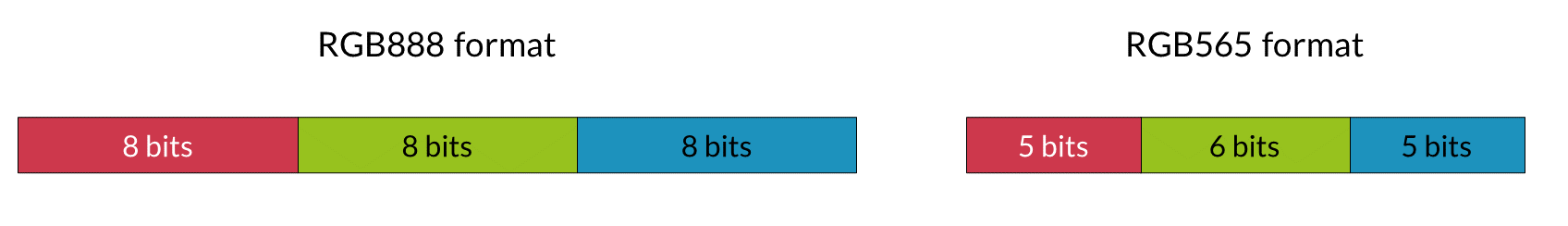However, Neon can still handle RGB565 data efficiently, and the vector shifts introduced in this section provide a method to do this.

#### Converting from RGB565 to RGB888

First, we consider converting RGB565 to RGB888. We assume that there are eight 16-bit pixels in register v0. We want to separate reds, greens, and blues into 8-bit elements across three registers v2 to v4.

The following code uses shift instructions to convert RGB565 to RGB888:

```ushr v1.16b, v0.16b, #3 // Shift red elements right by three bits,
// discarding the green bits at the bottom of
// the red 8-bit elements.

shrn v2.8b, v1.8h, #5   // Shift red elements right and narrow,
// discarding the blue and green bits.

shrn v3.8b, v0.8h, #5   // shift green elements right and narrow
// discarding the blue bits and some red bits
// due to narrowing.

shl v3.8b, v3.8b, #2    // shift green elements left, discarding the
// remaining red bits, and placing green bits
// in the correct place.

shl v0.16b, v0.16b, #3  // shift blue elements left to most significant
// bits of 8-bit color channel.

xtn v4.8b, v0.8h        // remove remaining red and green bits by
// narrowing to 8 bits.```

The effects of each instruction are described in the comments in the preceding code example. In summary, the operation that is performed on each channel is:

1. Remove color data for adjacent channels using shifts to push the bits off either end of the element.
2. Use a second shift to position the color data in the most significant bits of each element.
3. Perform narrowing to reduce the element size from 16-bits to 8-bits.
##### A small problem

You might notice that, if you use this code to convert to RGB888 format, the whites are not quite white. This is because, for each channel, the lowest two or three bits are zero, rather than one. A white represented in RGB565 as (`0x1F`, `0x3F`, `0x1F`) becomes (`0xF8`, `0xFC`, `0xF8`) in RGB888. This can be fixed using shift with insert to place some of the most significant bits into the lower bits.

#### Converting from RGB888 to RGB565

Now, we can look at the reverse operation, converting RGB888 format to RGB565. The RGB888 data is in the format that is produced by the preceding code. Data is separated across three registers v0 to v2, with each vector register containing eight elements of each color. The result is stored as eight 16-bit RGB565 elements in register v3.

The following code converts RGB888 data in registers v0, v1, and v2 to RGB565 data in v3:

```shll v3.8h, v0.8b, #8   // Shift red elements left to most significant
//  bits of wider 16-bit elements.

shll v4.8h, v1.8b, #8   // Shift green elements left to most significant
//  bits of wider 16-bit elements.

sri v3.8h, v4.8h, #5    // Shift green elements right and insert into
//  red elements.

shll v4.8h, v2.8b, #8   // Shift blue elements left to most significant
//  bits of wider 16-bit elements.

sri v3.8h, v4.8h, #11   // Shift blue elements right and insert into
//  red and green elements.```

Again, the detail is in the comments for each instruction in the preceding code, but the process for each channel is as follows:

1. Lengthen each element to 16 bits, and shift the color data into the most significant bits.
2. Use shift right with insert to position each color channel in the result register.

### Conclusion

The powerful range of shift instructions provided by Neon allows you to do the following:

• Quickly divide and multiply vectors by powers of two, with rounding and saturation.
• Shift and copy bits from one vector to another.
• Make interim calculations at high precision and accumulate results at a lower precision.

## Related information

Here are some resources related to material in this guide: